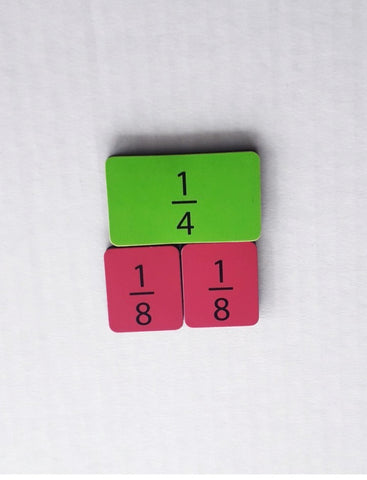Teaching Equivalent Fractions with Montessori

Equivalent Fractions are a complex topic that can be taught really well with the Montessori fraction equipment.

Prior to presenting this concept students will need to

• Be confident with their times tables
• Have worked with adding fractions for example know 1/6 + 1/6 = 2/6

The lesson

1) What is my name? Sarah. Yes that is my name. I am also called Sarah Smith. People sometimes also call me Sez (continue in this fashion) all those names are used for me.

2) Fractions have something similar. This is a quarter (present a quarter from the fraction circles)

3) There are two other fractions that if I put them together they will equal the same thing as a quarter. Present two eighths and place them on top of the quarter to show equivalence. So 2/8 is a different name for 1/44) Write for the students 1/4 = 2/8

5) There are other fractions like this too. Here is a half let's see what other names a half has. Let the children 'play' to work out what could equal a half. Have the students line up the fractions that equal a half beside each other.

6) Now get out a 'fraction wall' and have students place the fractions that equal a half underneath the half similar to the picture below. The fraction wall makes it clearer to students what fractions are equivalent compared to the Montessori fraction pieces.7) Students can now make  equivalent fraction booklets that show equivalents get your free download of the equivalent fraction booklets here8) With teacher made task cards the students can practise making equivalent fractions. Ensure the task cards have numbers that are not part of the fraction circles this will encourage students to use their multiplication and division knowledge.

Make sure to also check out

Teaching Improper fractions with Montessori

Teaching fractions with the Montessori stamp game

Interested in More Montessori Curriculum resource blog posts? Find them here

You can join my mailing list to get more Montessori resources, notifications of new products and news here